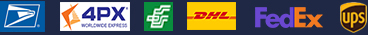•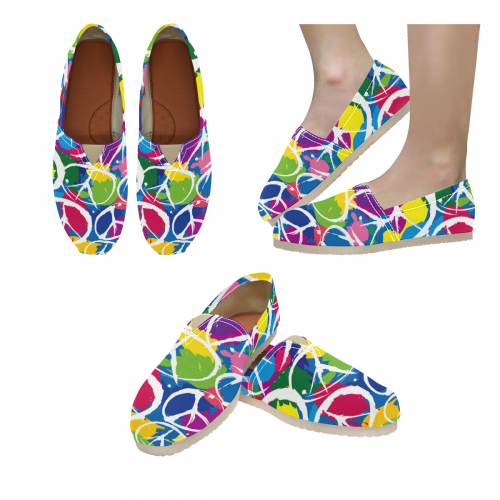•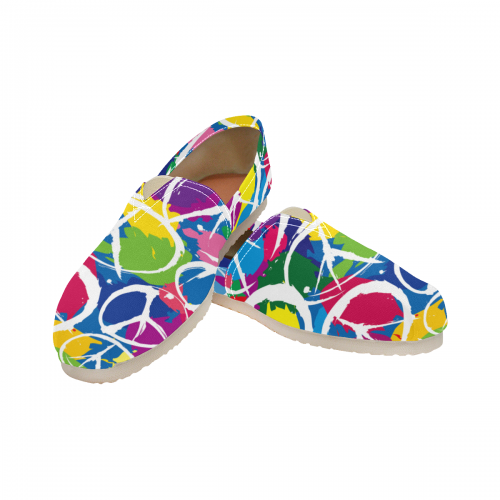•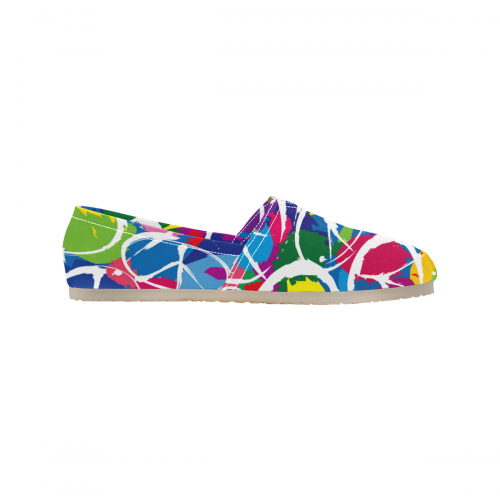•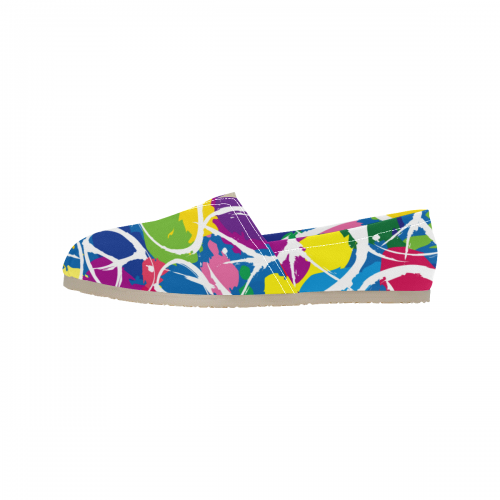### Women's Canvas Slip-On Shoes(Unisex)(Model1206)

\$23.99
Retail Price \$49.99

Partial Shipping Included

•   White

EUR35.5  =  WUS5-MUS3.5      = 227mm = 8.94inch
EUR36     =  WUS5.5-MUS4      = 231mm = 9.09inch
EUR36.5  =  WUS6- MUS4.5     = 235mm = 9.25inch
EUR37     =  WUS6.5-MUS5      = 239mm = 9.41inch
EUR38     =  WUS7-MUS5.5      = 243mm = 9.57inch
EUR39     =  WUS7.5-MUS6      = 248mm = 9.76nch
EUR39.5  =  WUS8 -MUS6.5     = 252mm = 9.92inch
EUR40     =  WUS8.5-MUS7      = 256mm = 10.08inch
EUR40.5  =  WUS9-MUS7.5      = 260mm = 10.24inch
EUR41     =  WUS9.5-MUS8      = 265mm = 10.43inch
EUR42     =  WUS10-MUS8.5    = 269mm = 10.59inch
EUR42.5  =  WUS10.5-MUS9    = 273mm = 10.75inch
EUR43     =  WUS11-MUS9.5    = 277mm = 10.91inch
EUR44     =  WUS11.5-MUS10  = 281mm = 11.06inch
EUR44.5  =  WUS12 -MUS10.5 = 286mm = 11.26inch
EUR45     =  WUS12.5-MUS11  = 290mm = 11.42inch
EUR45.5  =  WUS13-MUS11.5  = 294mm = 11.57inch
EUR46     =  WUS13.5-MUS12  = 300mm = 11.81inch
EUR47     =  WUS14.5-MUS13  = 307mm = 12.09inch

Payment methods we accept: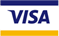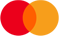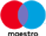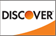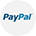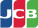We deliver with: